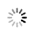# 使用r语言绘制正弦函数图像的代码

5月前 ⋅ 328 阅读
```以下是使用r语言绘制正弦函数图像的代码：

```{r}
# 生成x轴的数据
x <- seq(-2 * pi, 2 * pi, length.out = 200)

# 计算y轴的数据（正弦函数）
y <- sin(x)

# 绘制图像
plot(x, y, type = "l", col = "blue", lwd = 2, xlab = "x", ylab = "y", main = "Sin function")
```

- `seq(-2 * pi, 2 * pi, length.out = 200)` 生成了一组从 `-2π` 到 `2π` 的等间隔的 `x` 值，共有 `200` 个点。
- `sin(x)` 计算了每个点的 `y` 值，即正弦函数的值。
- `plot(x, y, type = "l", col = "blue", lwd = 2, xlab = "x", ylab = "y", main = "Sin function")` 绘制了图像。`type = "l"` 代表绘制一条连续的线，`col = "blue"` 和 `lwd = 2` 分别指定了线的颜色和宽度。`xlab` 和 `ylab` 分别指定了x轴和y轴的标签，`main` 指定了图像的标题。

### 相关推荐